Share

# HC Verma solutions for Class 12 Concepts of Physics 1 chapter 12 - Simple Harmonics Motion [Latest edition]

Textbook page

#### Chapters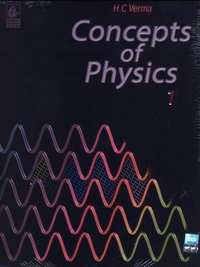## Chapter 12: Simple Harmonics Motion

#### HC Verma solutions for Class 12 Concepts of Physics 1 Chapter 12 Simple Harmonics Motion Exercise Short Answers [Pages 249 - 250]

Short Answers | Q 1 | Page 249

A person goes to bed at sharp 10.00 pm every day. Is it an example of periodic motion? If yes, what is the time period? If no, why?

Short Answers | Q 2 | Page 249

A particle executing simple harmonic motion comes to rest at the extreme positions. Is the resultant force on the particle zero at these positions according to Newton's first law?

Short Answers | Q 3 | Page 249

Can simple harmonic motion take place in a non-inertial frame? If yes, should the ratio of the force applied with the displacement be constant?

Short Answers | Q 4 | Page 249

A particle executes simple harmonic motion. If you are told that its velocity at this instant is zero, can you say what is its displacement? If you are told that its velocity at this instant is maximum, can you say what is its displacement?

Short Answers | Q 5 | Page 249

A small creature moves with constant speed in a vertical circle on a bright day. Does its shadow formed by the sun on a horizontal plane move in a sample harmonic motion?

Short Answers | Q 6 | Page 249

A particle executes simple harmonic motion Let P be a point near the mean position and Q be a point near an extreme. The speed of the particle at P is larger than the speed at Q. Still the particle crosses Pand Q equal number of times in a given time interval. Does it make you unhappy?

Short Answers | Q 7 | Page 250

In measuring time period of a pendulum, it is advised to measure the time between consecutive passage through the mean position in the same direction. This is said to result in better accuracy than measuring time between consecutive passage through an extreme position. Explain.

Short Answers | Q 8 | Page 250

It is proposed to move a particle in simple harmonic motion on a rough horizontal surface by applying an external force along the line of motion. Sketch the graph of the applied force against the position of the particle. Note that the applied force has two values for a given position depending on whether the particle is moving in positive or negative direction.

Short Answers | Q 9 | Page 250

Can the potential energy in a simple harmonic motion be negative? Will it be so if we choose zero potential energy at some point other than the mean position?

Short Answers | Q 10 | Page 250

The energy of system in simple harmonic motion is given by $E = \frac{1}{2}m \omega^2 A^2 .$ Which of the following two statements is more appropriate?
(A) The energy is increased because the amplitude is increased.
(B) The amplitude is increased because the energy is increased.

Short Answers | Q 11 | Page 250

A pendulum clock gives correct time at the equator. Will it gain time or loose time as it is taken to the poles?

Short Answers | Q 12 | Page 250

Can a pendulum clock be used in an earth-satellite?

Short Answers | Q 13 | Page 250

A hollow sphere filled with water is used as the bob of a pendulum. Assume that the equation for simple pendulum is valid with the distance between the point of suspension and centre of mass of the bob acting as the effective length of the pendulum. If water slowly leaks out of the bob, how will the time period vary?

Short Answers | Q 14 | Page 250

A block of known mass is suspended from a fixed support through a light spring. Can you find the time period of vertical oscillation only by measuring the extension of the spring when the block is in equilibrium?

Short Answers | Q 15 | Page 250

A platoon of soldiers marches on a road in steps according to the sound of a marching band. The band is stopped and the soldiers are ordered to break the steps while crossing a bridge. Why?

Short Answers | Q 16 | Page 250

The force acting on a particle moving along X-axis is F = −k(x − vo t) where k is a positive constant. An observer moving at a constant velocity v0 along the X-axis looks at the particle. What kind of motion does he find for the particle?

#### HC Verma solutions for Class 12 Concepts of Physics 1 Chapter 12 Simple Harmonics Motion Exercise MCQ [Pages 250 - 251]

MCQ | Q 1 | Page 250

A student says that he had applied a force $F = - k\sqrt{x}$ on a particle and the particle moved in simple harmonic motion. He refuses to tell whether k is a constant or not. Assume that he was worked only with positive x and no other force acted on the particle.

• As x increases k increases.

• As x increases k decreases.

• As x increases k remains constant.

• The motion cannot be simple harmonic.

MCQ | Q 2 | Page 250

The time period of a particle in simple harmonic motion is equal to the time between consecutive appearances of the particle at a particular point in its motion. This point is

• the mean position

• an extreme position

• between the mean position and the positive extreme

• between the mean position and the negative extreme

MCQ | Q 3 | Page 250

The time period of a particle in simple harmonic motion is equal to the smallest time between the particle acquiring a particular velocity $\vec{v}$ . The value of v is

• vmax

• 0

• between 0 and vmax

• between 0 and −vmax

MCQ | Q 4 | Page 250

The displacement of a particle in simple harmonic motion in one time period is

• A

• 2A

• 4A

• zero

MCQ | Q 5 | Page 250

The distance moved by a particle in simple harmonic motion in one time period is

• A

• 2A

• 4A

• zero

MCQ | Q 6 | Page 250

The average acceleration in one time period in a simple harmonic motion is

• Aω2

• Aω2/2

• $A \omega^2 /\sqrt{2}$

• zero

MCQ | Q 7 | Page 250

The motion of a particle is given by x = A sin ωt + B cos ωt. The motion of the particle is

• not simple harmonic

• simple harmonic with amplitude A + B

• simple harmonic with amplitude (A + B)/2

• simple harmonic with amplitude

MCQ | Q 8 | Page 250

The displacement of a particle is given by $\overrightarrow{r} = A\left( \overrightarrow{i} \cos\omega t + \overrightarrow{j} \sin\omega t \right) .$ The motion of the particle is

• simple harmonic

• on a straight line

• on a circle

• with constant acceleration

MCQ | Q 9 | Page 250

A particle moves on the X-axis according to the equation x = A + B sin ωt. The motion is simple harmonic with amplitude

• A

• B

• A + B

• $\sqrt{A^2 + B^2} .$

MCQ | Q 10 | Page 250

Figure represents two simple harmonic motions.

The parameter which has different values in the two motions is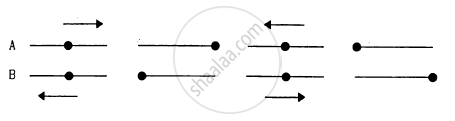• amplitude

• frequency

• phase

• maximum velocity

MCQ | Q 11 | Page 251

The total mechanical energy of a spring-mass system in simple harmonic motion is $E = \frac{1}{2}m \omega^2 A^2 .$ Suppose the oscillating particle is replaced by another particle of double the mass while the amplitude A remains the same. The new mechanical energy will

• become 2E

• become E/2

• become $\sqrt{2}E$

• remain E

MCQ | Q 12 | Page 251

The average energy in one time period in simple harmonic motion is

• $\frac{1}{2}m \omega^2 A^2$

• $\frac{1}{4}m \omega^2 A^2$

• m ω2A2

• zero

MCQ | Q 13 | Page 251

A particle executes simple harmonic motion with a frequency v. The frequency with which the kinetic energy oscillates is

• v/2

• v

• (c) 2 v

• zero

MCQ | Q 14 | Page 251

A particle executes simple harmonic motion under the restoring force provided by a spring. The time period is T. If the spring is divided in two equal parts and one part is used to continue the simple harmonic motion, the time period will

• remain T

• become 2T

• become T/2

• become $T/\sqrt{2}$

MCQ | Q 15 | Page 251

Two bodies A and B of equal mass are suspended from two separate massless springs of spring constant k1 and k2 respectively. If the bodies oscillate vertically such that their maximum velocities are equal, the ratio of the amplitude of A to that of B is

• k1/k2

• $\sqrt{k_1 / k_2}$

• k2/k1

• $\sqrt{k_2 / k_1}$

MCQ | Q 16 | Page 251

A spring-mass system oscillates with a frequency v. If it is taken in an elevator slowly accelerating upward, the frequency will

• increase

• decrease

• remain same

• become zero

MCQ | Q 17 | Page 251

A spring-mass system oscillates with a car. If the car accelerates on a horizontal road, the frequency of oscillation will

• increase

• decrease

• remain same

• become zero

MCQ | Q 18 | Page 251

A pendulum clock that keeps correct time on the earth is taken to the moon. It will run

• at correct rate

• 6 times faster

• $\sqrt{6}$ times faster

• $\sqrt{6}$ times slower

MCQ | Q 19 | Page 251

A wall clock uses a vertical spring-mass system to measure the time. Each time the mass reaches an extreme position, the clock advances by a second. The clock gives correct time at the equator. If the clock is taken to the poles it will

• run slow

• run fast

• stop working

• give correct time

MCQ | Q 20 | Page 251

A pendulum clock keeping correct time is taken to high altitudes,

• it will keep correct time

• its length should be increased to keep correct time

• its length should be decreased to keep correct time

• it cannot keep correct time even if the length is changed

MCQ | Q 21 | Page 251

The free end of a simple pendulum is attached to the ceiling of a box. The box is taken to a height and the pendulum is oscillated. When the bob is at its lowest point, the box is released to fall freely. As seen from the box during this period, the bob will

• continue its oscillation as before

• stop

• will go in a circular path

• move on a straight line

#### HC Verma solutions for Class 12 Concepts of Physics 1 Chapter 12 Simple Harmonics Motion Exercise MCQ [Pages 15 - 252]

MCQ | Q 1 | Page 251

Select the correct statements.
(a) A simple harmonic motion is necessarily periodic.
(b) A simple harmonic motion is necessarily oscillatory.
(c) An oscillatory motion is necessarily periodic.
(d) A periodic motion is necessarily oscillatory.

MCQ | Q 2 | Page 251

A particle moves in a circular path with a uniform speed. Its motion is

• periodic

• oscillatory

• simple harmonic

• angular simple harmonic

MCQ | Q 3 | Page 251

A particle is fastened at the end of a string and is whirled in a vertical circle with the other end of the string being fixed. The motion of the particle is

• periodic

• oscillatory

• simple harmonic

• angular simple harmonic

MCQ | Q 4 | Page 251

A particle moves in a circular path with a continuously increasing speed. Its motion is

• periodic

• oscillatory

• simple harmonic

• none of them

MCQ | Q 5 | Page 251

The motion of a torsional pendulum is
(a) periodic
(b) oscillatory
(c) simple harmonic
(d) angular simple harmonic

MCQ | Q 6 | Page 251

Which of the following quantities are always negative in a simple harmonic motion?

(a) $\vec{F} . \vec{a} .$

(b) $\vec{v} . \vec{r} .$

(c) $\vec{a} . \vec{r} .$

(d)$\vec{F} . \vec{r} .$

MCQ | Q 7 | Page 251

Which of the following quantities are always positive in a simple harmonic motion?

• $\vec{F} . \vec{a} .$

• $\vec{v} . \vec{r} .$

• $\vec{a} . \vec{r} .$

• $\vec{F} . \vec{r} .$

MCQ | Q 8 | Page 251

Which of the following quantities are always zero in a simple harmonic motion?
(a) $\vec{F} \times \vec{a} .$

(b) $\vec{v} \times \vec{r} .$

(c) $\vec{a} \times \vec{r} .$

(d) $\vec{F} \times \vec{r} .$

MCQ | Q 9 | Page 251

Suppose a tunnel is dug along a diameter of the earth. A particle is dropped from a point, a distance h directly above the tunnel. The motion of the particle as seen from the earth is
(a) simple harmonic
(b) parabolic
(c) on a straight line
(d) periodic

MCQ | Q 10 | Page 252

For a particle executing simple harmonic motion, the acceleration is proportional to

• displacement from the mean position

• distance from the mean position

• distance travelled since t = 0

• speed

MCQ | Q 11 | Page 252

A particle moves in the X-Y plane according to the equation $\overrightarrow{r} = \left( \overrightarrow{i} + 2 \overrightarrow{j} \right)A\cos\omega t .$

The motion of the particle is
(a) on a straight line
(b) on an ellipse
(c) periodic
(d) simple harmonic

MCQ | Q 12 | Page 252

A particle moves on the X-axis according to the equation x = x0 sin2 ωt. The motion is simple harmonic

• with amplitude x0

• with amplitude 2x0

• with time period $\frac{2\pi}{\omega}$

• with time period $\frac{\pi}{\omega} .$

MCQ | Q 13 | Page 252

In a simple harmonic motion

•  the potential energy is always equal to the kinetic energy

• the potential energy is never equal to the kinetic energy

• the average potential energy in any time interval is equal to the average kinetic energy in that time interval

• the average potential energy in one time period is equal to the average kinetic energy in this period.

MCQ | Q 14 | Page 252

In a simple harmonic motion
(a) the maximum potential energy equals the maximum kinetic energy
(b) the minimum potential energy equals the minimum kinetic energy
(c) the minimum potential energy equals the maximum kinetic energy
(d) the maximum potential energy equals the minimum kinetic energy

MCQ | Q 15 | Page 15

An object is released from rest. The time it takes to fall through a distance h and the speed of the object as it falls through this distance are measured with a pendulum clock. The entire apparatus is taken on the moon and the experiment is repeated
(a) the measured times are same
(b) the measured speeds are same
(c) the actual times in the fall are equal
(d) the actual speeds are equal

MCQ | Q 16 | Page 252

Which of the following will change the time period as they are taken to moon?
(a) A simple pendulum
(b) A physical pendulum
(c) A torsional pendulum
(d) A spring-mass system

#### HC Verma solutions for Class 12 Concepts of Physics 1 Chapter 12 Simple Harmonics Motion [Pages 252 - 256]

Q 1 | Page 252

A particle executes simple harmonic motion with an amplitude of 10 cm and time period 6 s. At t = 0 it is at position x = 5 cm going towards positive x-direction. Write the equation for the displacement x at time t. Find the magnitude of the acceleration of the particle at t = 4 s.

Q 2 | Page 252

The position, velocity and acceleration of a particle executing simple harmonic motion are found to have magnitude 2 cm, 1 m s−1 and 10 m s−2 at a certain instant. Find the amplitude and the time period of the motion.

Q 3 | Page 252

A particle executes simple harmonic motion with an amplitude of 10 cm. At what distance from the mean position are the kinetic and potential energies equal?

Q 4 | Page 252

The maximum speed and acceleration of a particle executing simple harmonic motion are 10 cm/s and 50 cm/s2. Find the position(s) of the particle when the speed is 8 cm/s.

Q 5 | Page 252

A particle having mass 10 g oscillates according to the equation x = (2.0 cm) sin [(100 s−1)t + π/6]. Find (a) the amplitude, the time period and the spring constant. (c) the position, the velocity and the acceleration at t = 0.

Q 6 | Page 252

The equation of motion of a particle started at t = 0 is given by x = 5 sin (20t + π/3), where x is in centimetre and in second. When does the particle
(a) first come to rest
(b) first have zero acceleration
(c) first have maximum speed?

Q 7 | Page 252

Consider a particle moving in simple harmonic motion according to the equation x = 2.0 cos (50 πt + tan−1 0.75) where x is in centimetre and t in second. The motion is started at t = 0. (a) When does the particle come to rest for the first time? (b) When does he acceleration have its maximum magnitude for the first time? (c) When does the particle come to rest for the second time ?

Q 8 | Page 252

Consider a simple harmonic motion of time period T. Calculate the time taken for the displacement to change value from half the amplitude to the amplitude.

Q 9 | Page 252

The pendulum of a clock is replaced by a spring-mass system with the spring having spring constant 0.1 N/m. What mass should be attached to the spring?

Q 10 | Page 252

A block suspended from a vertical spring is in equilibrium. Show that the extension of the spring equals the length of an equivalent simple pendulum, i.e., a pendulum having frequency same as that of the block.

Q 11 | Page 252

A block of mass 0.5 kg hanging from a vertical spring executes simple harmonic motion of amplitude 0.1 m and time period 0.314 s. Find the maximum force exerted by the spring on the block.

Q 12 | Page 252

A body of mass 2 kg suspended through a vertical spring executes simple harmonic motion of period 4 s. If the oscillations are stopped and the body hangs in equilibrium find the potential energy stored in the spring.

Q 13 | Page 253

A spring stores 5 J of energy when stretched by 25 cm. It is kept vertical with the lower end fixed. A block fastened to its other end is made to undergo small oscillations. If the block makes 5 oscillations each second what is the mass of the block?

Q 14 | Page 253

A small block of mass m is kept on a bigger block of mass M which is attached to a vertical spring of spring constant k as shown in the figure. The system oscillates vertically. (a) Find the resultant force on the smaller block when it is displaced through a distance x above its equilibrium position. (b) Find the normal force on the smaller block at this position. When is this force smallest in magnitude? (c) What can be the maximum amplitude with which the two blocks may oscillate together?Q 15 | Page 253

The block of mass m1 shown in figure is fastened to the spring and the block of mass m2 is placed against it. (a) Find the compression of the spring in the equilibrium position. (b) The blocks are pushed a further distance (2/k) (m1 + m2)g sin θ against the spring and released. Find the position where the two blocks separate. (c) What is the common speed of blocks at the time of separation?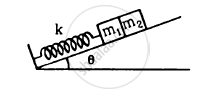Q 16 | Page 253

In following figure k = 100 N/m M = 1 kg and F = 10 N. (a) Find the compression of the spring in the equilibrium position. (b) A sharp blow by some external agent imparts a speed of 2 m/s to the block towards left. Find the sum of the potential energy of the spring and the kinetic energy of the block at this instant. (c) Find the time period of the resulting simple harmonic motion. (d) Find the amplitude. (e) Write the potential energy of the spring when the block is at the left extreme. (f) Write the potential energy of the spring when the block is at the right extreme.
The answer of (b), (e) and (f) are different. Explain why this does not violate the principle of conservation of energy.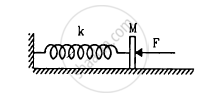Q 17 | Page 253

Find the time period of the oscillation of mass m in figures  a, b, c. What is the equivalent spring constant of the pair of springs in each case?]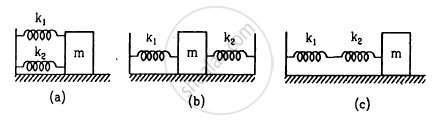Q 18 | Page 253

The spring shown in figure is unstretched when a man starts pulling on the cord. The mass of the block is M. If the man exerts a constant force F, find (a) the amplitude and the time period of the motion of the block, (b) the energy stored in the spring when the block passes through the equilibrium position and (c) the kinetic energy of the block at this position.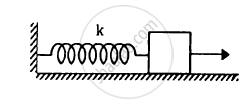Q 19 | Page 253

A particle of mass m is attatched to three springs A, B and C of equal force constants kas shown in figure . If the particle is pushed slightly against the spring C and released, find the time period of oscillation.Q 20 | Page 253

Repeat the previous exercise if the angle between each pair of springs is 120° initially.

Q 21 | Page 253

The springs shown in the figure are all unstretched in the beginning when a man starts pulling the block. The man exerts a constant force F on the block. Find the amplitude and the frequency of the motion of the block.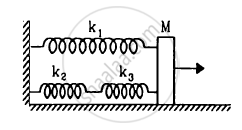Q 22 | Page 253

Find the elastic potential energy stored in each spring shown in figure, when the block is in equilibrium. Also find the time period of vertical oscillation of the block.

Q 22 | Page 253

Find the elastic potential energy stored in each spring shown in figure when the block is in equilibrium. Also find the time period of vertical oscillation of the block.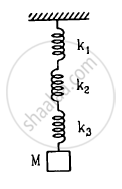Q 23 | Page 254

The string the spring and the pulley shown in figure are light. Find the time period of the mass m.Q 24 | Page 254

Solve the previous problem if the pulley has a moment of inertia I about its axis and the string does not slip over it.

Q 25 | Page 254

Consider the situation shown in figure . Show that if the blocks are displaced slightly in opposite direction and released, they will execute simple harmonic motion. Calculate the time period.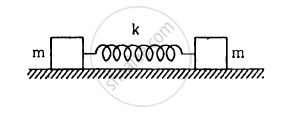Q 26 | Page 254

A rectangle plate of sides a and b is suspended from a ceiling by two parallel string of length L each in Figure . The separation between the string is d. The plate is displaced slightly in its plane keeping the strings tight. Show that it will execute simple harmonic motion. Find the time period.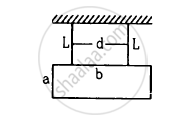Q 27 | Page 254

A 1 kg block is executing simple harmonic motion of amplitude 0.1 m on a smooth horizontal surface under the restoring force of a spring of spring constant 100 N/m. A block of mass 3 kg is gently placed on it at the instant it passes through the mean position. Assuming that the two blocks move together, find the frequency and the amplitude of the motion.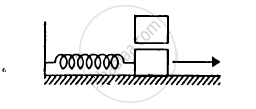Q 28 | Page 254

The left block in figure moves at a speed v towards the right block placed in equilibrium. All collisions to take place are elastic and the surfaces are frictionless. Show that the motions of the two blocks are periodic. Find the time period of these periodic motions. Neglect the widths of the blocks.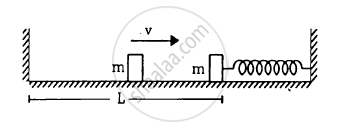Q 29 | Page 254

Find the time period of the motion of the particle shown in figure . Neglect the small effect of the bend near the bottom.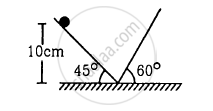Q 30 | Page 254

All the surfaces shown in figure are frictionless. The mass of the care is M, that of the block is m and the spring has spring constant k. Initially the car and the block are at rest and the spring is stretched through a length x0 when the system is released. (a) Find the amplitudes of the simple harmonic motion of the block and of the care as seen from the road. (b) Find the time period(s) of the two simple harmonic motions.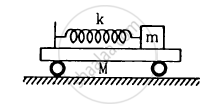Q 31 | Page 254

A uniform plate of mass M stays horizontally and symmetrically on two wheels rotating in opposite direction in Figure . The separation between the wheels is L. The friction coefficient between each wheel and the plate is μ. Find the time period of oscillation of the plate if it is slightly displaced along its length and released.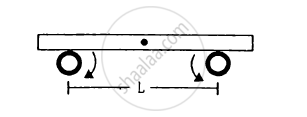Q 32 | Page 254

A pendulum having time period equal to two seconds is called a seconds pendulum. Those used in pendulum clocks are of this type. Find the length of a second pendulum at a place where = π2 m/s2.

Q 33 | Page 254

The angle made by the string of a simple pendulum with the vertical depends on time as $\theta = \frac{\pi}{90} \sin \left[ \left( \pi s^{- 1} \right)t \right]$ .Find the length of the pendulum if g = π2 m2.

Q 34 | Page 255

The pendulum of a certain clock has time period 2.04 s. How fast or slow does the clock run during 24 hours?

Q 35 | Page 255

A pendulum clock giving correct time at a place where g = 9.800 m/s2 is taken to another place where it loses 24 seconds during 24 hours. Find the value of g at this new place.

Q 36 | Page 255

A simple pendulum is constructed by hanging a heavy ball by a 5.0 m long string. It undergoes small oscillations. (a) How many oscillations does it make per second? (b) What will be the frequency if the system is taken on the moon where acceleration due to gravitation of the moon is 1.67 m/s2?

Q 37 | Page 255

A small block oscillates back and forth on a smooth concave surface of radius R ib Figure . Find the time period of small oscillation.

Q 38 | Page 255

A small block oscillates back and forth on a smooth concave surface of radius R in Figure. Find the time period of small oscillation.Q 39 | Page 255

A spherical ball of mass m and radius r rolls without slipping on a rough concave surface of large radius R. It makes small oscillations about the lowest point. Find the time period.

Q 40 | Page 255

A simple pendulum of length 40 cm is taken inside a deep mine. Assume for the time being that the mine is 1600 km deep. Calculate the time period of the pendulum there. Radius of the earth = 6400 km.

Q 41 | Page 255

Assume that a tunnel is dug across the earth (radius = R) passing through its centre. Find the time a particle takes to cover the length of the tunnel if (a) it is projected into the tunnel with a speed of $\sqrt{gR}$ (b) it is released from a height R above the tunnel (c) it is thrown vertically upward along the length of tunnel with a speed of $\sqrt{gR}$

Q 42 | Page 255

Assume that a tunnel is dug along a chord of the earth, at a perpendicular distance R/2 from the earth's centre where R is the radius of the earth. The wall of the tunnel is frictionless. (a) Find the gravitational force exerted by the earth on a particle of mass mplaced in the tunnel at a distance x from the centre of the tunnel. (b) Find the component of this force along the tunnel and perpendicular to the tunnel. (c) Find the normal force exerted by the wall on the particle. (d) Find the resultant force on the particle. (e) Show that the motion of the particle in the tunnel is simple harmonic and find the time period.

Q 43 | Page 255

A simple pendulum of length l is suspended through the ceiling of an elevator. Find the time period of small oscillations if the elevator (a) is going up with and acceleration a0(b) is going down with an acceleration a0 and (c) is moving with a uniform velocity.

Q 44 | Page 255

A simple pendulum of length 1 feet suspended from the ceiling of an elevator takes π/3 seconds to complete one oscillation. Find the acceleration of the elevator.

Q 45 | Page 255

A simple pendulum fixed in a car has a time period of 4 seconds when the car is moving uniformly on a horizontal road. When the accelerator is pressed, the time period changes to 3.99 seconds. Making an approximate analysis, find the acceleration of the car.

Q 46 | Page 255

A simple pendulum of length l is suspended from the ceiling of a car moving with a speed v on a circular horizontal road of radius r. (a) Find the tension in the string when it is at rest with respect to the car. (b) Find the time period of small oscillation.

Q 47 | Page 255

The ear-ring of a lady shown in figure has a 3 cm long light suspension wire. (a) Find the time period of small oscillations if the lady is standing on the ground. (b) The lady now sits in a merry-go-round moving at 4 m/s1 in a circle of radius 2 m. Find the time period of small oscillations of the ear-ring.Q 48 | Page 255

Find the time period of small oscillations of the following systems. (a) A metre stick suspended through the 20 cm mark. (b) A ring of mass m and radius r suspended through a point on its periphery. (c) A uniform square plate of edge a suspended through a corner. (d) A uniform disc of mass m and radius r suspended through a point r/2 away from the centre.

Q 49 | Page 255

A uniform rod of length l is suspended by an end and is made to undergo small oscillations. Find the length of the simple pendulum having the time period equal to that of the road.

Q 50 | Page 255

A uniform disc of radius r is to be suspended through a small hole made in the disc. Find the minimum possible time period of the disc for small oscillations. What should be the distance of the hole from the centre for it to have minimum time period?

Q 51 | Page 255

A hollow sphere of radius 2 cm is attached to an 18 cm long thread to make a pendulum. Find the time period of oscillation of this pendulum. How does it differ from the time period calculated using the formula for a simple pendulum?

Q 52 | Page 255

A closed circular wire hung on a nail in a wall undergoes small oscillations of amplitude 20 and time period 2 s. Find (a) the radius of the circular wire, (b) the speed of the particle farthest away from the point of suspension as it goes through its mean position, (c) the acceleration of this particle as it goes through its mean position and (d) the acceleration of this particle when it is at an extreme position. Take g = π2 m/s2.

Q 53 | Page 255

A uniform disc of mass m and radius r is suspended through a wire attached to its centre. If the time period of the torsional oscillations be T, what is the torsional constant of the wire?

Q 54 | Page 256

Two small balls, each of mass m are connected by a light rigid rod of length L. The system is suspended from its centre by a thin wire of torsional constant k. The rod is rotated about the wire through an angle θ0 and released. Find the force exerted by the rod on one of the balls as the system passes through the mean position.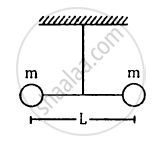Q 55 | Page 256

A particle is subjected to two simple harmonic motions of same time period in the same direction. The amplitude of the first motion is 3.0 cm and that of the second is 4.0 cm. Find the resultant amplitude if the phase difference between the motions is (a) 0°, (b) 60°, (c) 90°.

Q 56 | Page 256

Three simple harmonic motions of equal amplitude A and equal time periods in the same direction combine. The phase of the second motion is 60° ahead of the first and the phase of the third motion is 60° ahead of the second. Find the amplitude of the resultant motion.

Q 57 | Page 256

A particle is subjected to two simple harmonic motions given by x1 = 2.0 sin (100π t) and x2 = 2.0 sin (120 π t + π/3), where x is in centimeter and t in second. Find the displacement of the particle at (a) = 0.0125, (b) t = 0.025.

Q 58 | Page 256

A particle is subjected to two simple harmonic motions, one along the X-axis and the other on a line making an angle of 45° with the X-axis. The two motions are given by x = x0 sin ωt and s = s0 sin ωt. Find the amplitude of the resultant motion.

## Chapter 12: Simple Harmonics Motion## HC Verma solutions for Class 12 Concepts of Physics 1 chapter 12 - Simple Harmonics Motion

HC Verma solutions for Class 12 Concepts of Physics 1 chapter 12 (Simple Harmonics Motion) include all questions with solution and detail explanation. This will clear students doubts about any question and improve application skills while preparing for board exams. The detailed, step-by-step solutions will help you understand the concepts better and clear your confusions, if any. Shaalaa.com has the CBSE Class 12 Concepts of Physics 1 solutions in a manner that help students grasp basic concepts better and faster.

Further, we at Shaalaa.com provide such solutions so that students can prepare for written exams. HC Verma textbook solutions can be a core help for self-study and acts as a perfect self-help guidance for students.

Concepts covered in Class 12 Concepts of Physics 1 chapter 12 Simple Harmonics Motion are Force Law for Simple Harmonic Motion, Energy in Simple Harmonic Motion, Some Systems Executing Simple Harmonic Motion, Damped Simple Harmonic Motion, Forced Oscillations and Resonance, Displacement as a Function of Time, Periodic Functions, Oscillations - Frequency, Periodic and Oscillatory Motions, Simple Harmonic Motion, Simple Harmonic Motion and Uniform Circular Motion, Velocity and Acceleration in Simple Harmonic Motion.

Using HC Verma Class 12 solutions Simple Harmonics Motion exercise by students are an easy way to prepare for the exams, as they involve solutions arranged chapter-wise also page wise. The questions involved in HC Verma Solutions are important questions that can be asked in the final exam. Maximum students of CBSE Class 12 prefer HC Verma Textbook Solutions to score more in exam.

Get the free view of chapter 12 Simple Harmonics Motion Class 12 extra questions for Class 12 Concepts of Physics 1 and can use Shaalaa.com to keep it handy for your exam preparation